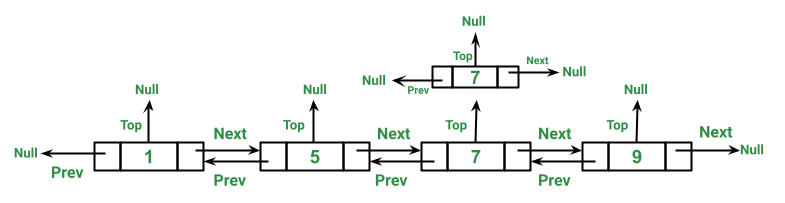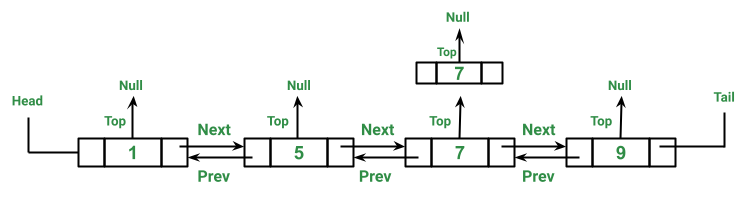# Java Program to Implement Triply Linked List

• Difficulty Level : Hard
• Last Updated : 13 Aug, 2021

Unlike arrays, linked list elements are not stored at a contiguous location; the elements are linked using pointers. In this post, methods to insert a new node in a linked list are discussed. A node can be inserted in three ways either at the front of the linked list or after a given node or at the end of the linked list. As we have already discussed Doubly Linked List (DLL) does contain an extra pointer, typically called the previous pointer, together with the next pointer and data which are there in a singly linked list.

Similarly, a  Triply Linked List (TLL) contains an extra pointer, typically called the top pointer, together with the next pointer, previous, and data which are there in the doubly linked list. The extra pointer here called as the top can be used for various purposes. For example, storing equal values on the same level. Refer to the below image for a better understanding. In this article, we will be inserting nodes in the linked list in sorted order. And we will be storing equal elements on the same level meaning they will be accessed by the top pointer.Illustration: Representation of a DLL node

```// Class for Triply Linked List
public class TLL {

class Node {
int data;
Node prev;
Node next;
Node top;
}

Node head = null, tail = null;

// To keep track of the number
// of nodes
int node_count = 0;
}```

Procedure:

1. Inserting a new node

Since we are storing nodes in a sorted order that’s why we have to find the correct position of the given node in the linked list.

1. If there are no nodes in the list ( List is empty ), then just make the head and tail point to this node.
2. If the given node is less than the head node, then just insert the node at the beginning.
3. If the given node is not less than the head node, then traverse the list and find the first node that is greater than the given node.
• If such a node doesn’t exist this means that the given node is greater than all the nodes. So insert it to the end of the List.
• If such a node does exist then insert the given node before the found node.
• If the given node is equal to some already present node then insert the given node to the top of the List.

2(A): Traverse the List from Head where we start from the head and keep going to the next node. If the top of the current node is not empty then print the top node first and then continue traversing the rest of the list.

2(B): Traverse the List from Tail or reverse traversal where we start from the tail and keep going to the previous node. If the top of the current node is not empty then print the top node first and then continue traversing the rest of the list.

Example:

## Java

 `// Java Program to Implement Triply Linked List` `// Importing all utility classes``import` `java.util.*;` `// Main Class``public` `class` `GFG {` `    ``// First let us create a Node class``    ``class` `Node {` `        ``// Data refers to the value in node``        ``int` `data;` `        ``// Being triply linked list,``        ``// three pointers needs to be defined``        ``Node prev;``        ``Node next;``        ``Node top;` `        ``// Parameterized constructor of Node class``        ``// to initialize the node whenever created``        ``Node(``int` `data)``        ``{` `            ``// this keyword refers to current object itself``            ``this``.data = data;` `            ``// Initializing all 3 pointers to null``            ``prev = ``null``;``            ``next = ``null``;``            ``top = ``null``;``        ``}``    ``}` `    ``// Defining two new pointers for iterate over to perform``    ``// operations over the triply linked list Head and Tail``    ``// pointer``    ``Node head = ``null``, tail = ``null``;` `    ``// Declaring and initializing variable to``    ``// keep track of the number of nodes``    ``int` `node_count = ``0``;` `    ``// Method 1``    ``// To insert a new node``    ``public` `void` `insert(``int` `new_data)``    ``{` `        ``// Create new node with the given data``        ``Node new_node = ``new` `Node(new_data);` `        ``// curr_node to traverse the List``        ``Node curr_node = ``null``;` `        ``// If List is empty then``        ``// make head and tail``        ``// equal to this node``        ``if` `(node_count == ``0``) {` `            ``// Case 1: Of LinkedList is empty``            ``// If there is no such node existing``            ``tail = new_node;``            ``head = new_node;``            ``curr_node = head;` `            ``// So next will be assigned a null``            ``curr_node.next = ``null``;``            ``curr_node.prev = ``null``;``            ``curr_node.top = ``null``;` `            ``// Increment the node count``            ``node_count++;``        ``}` `        ``// Case 2: If LinkedList is not empty` `        ``// Case 2(A): If given node is less then the head``        ``else` `{` `            ``// Make curr_node point to head``            ``curr_node = head;` `            ``// If given node is less then the head``            ``// insert at the beginning``            ``if` `(new_node.data < curr_node.data) {` `                ``// Linking two nodes through addresses``                ``new_node.next = curr_node;``                ``curr_node.prev = new_node;``                ``new_node.prev = ``null``;``                ``head = new_node;``                ``curr_node = head;` `                ``// Adjusting the tail``                ``do` `{` `                    ``curr_node = curr_node.next;``                ``} ``while` `(curr_node.next != ``null``);``                ``tail = curr_node;``            ``}` `            ``// CAse 2(B): If given node is not less then the``            ``// head``            ``else` `{` `                ``// last_node to keep track of``                ``// the last visited node``                ``Node last_node = curr_node;` `                ``// Goal is to traverse the List and``                ``// find first node greater than new_node``                ``while` `(curr_node != ``null``                       ``&& new_node.data > curr_node.data) {``                    ``last_node = curr_node;``                    ``curr_node = curr_node.next;` `                    ``// If curr_node is null that``                    ``// means we have reached the``                    ``// end of the list so insert the``                    ``// node at the end and update the tail``                    ``if` `(curr_node == ``null``) {` `                        ``last_node.next = new_node;``                        ``new_node.prev = last_node;``                        ``new_node.next = ``null``;``                        ``tail = new_node;` `                        ``// Haulting the execution of the``                        ``// program using break keyword``                        ``break``;``                    ``}` `                    ``else` `if` `(new_node.data``                             ``<= curr_node.data) {` `                        ``// If curr_node is greater than``                        ``// the new_node then insert the``                        ``// new_node before curr_nod and``                        ``// update the tail``                        ``if` `(new_node.data``                            ``< curr_node.data) {` `                            ``last_node.next = new_node;``                            ``new_node.prev = last_node;``                            ``new_node.next = curr_node;``                            ``curr_node.prev = new_node;``                            ``if` `(curr_node.next != ``null``) {` `                                ``do` `{` `                                    ``curr_node``                                        ``= curr_node.next;``                                ``}` `                                ``while` `(curr_node.next``                                       ``!= ``null``);``                            ``}` `                            ``tail = curr_node;` `                            ``break``;``                        ``}` `                        ``// If curr_node is equal to the``                        ``// new_node then insert the``                        ``// new_node to the top of the``                        ``// curr_nod and update the tail``                        ``else` `if` `(curr_node.data``                                 ``== new_node.data) {``                            ``last_node = curr_node;``                            ``while` `(last_node.top != ``null``) {` `                                ``last_node = last_node.top;``                            ``}` `                            ``last_node.top = new_node;``                            ``new_node.top = ``null``;` `                            ``break``;``                        ``}``                    ``}``                ``}``            ``}``        ``}``    ``}` `    ``// Method 2``    ``// Traversing list from head``    ``public` `void` `traverse_head()``    ``{` `        ``Node node = head;``        ``Node curr = ``null``;` `        ``while` `(node != ``null``) {``            ``System.out.print(node.data + ``"\t"``);``            ``curr = node;` `            ``// If curr has top node``            ``// then traverse them first``            ``while` `(curr.top != ``null``) {``                ``curr = curr.top;` `                ``// Print top node first followed by rest of``                ``// list``                ``System.out.print(``"top->"` `+ curr.data``                                 ``+ ``"\t"``);``            ``}` `            ``// Update the node to next node``            ``node = node.next;``        ``}` `        ``// New line``        ``System.out.println();``    ``}` `    ``// Method 3``    ``// Traversing list from tail``    ``public` `void` `traverse_tail()``    ``{` `        ``Node node = tail;``        ``Node curr = ``null``;` `        ``while` `(node != ``null``) {` `            ``System.out.print(node.data + ``"\t"``);``            ``curr = node;` `            ``// If curr has top node``            ``// then traverse them first``            ``while` `(curr.top != ``null``) {` `                ``curr = curr.top;` `                ``// Print top node first followed by rest of``                ``// list``                ``System.out.print(``"top->"` `+ curr.data``                                 ``+ ``"\t"``);``            ``}` `            ``// Update the node to prev node``            ``node = node.prev;``        ``}` `        ``// New line``        ``System.out.println();``    ``}` `    ``// Method 4``    ``// Main driver method``    ``public` `static` `void` `main(String args[])``    ``{``        ``// Creating an object of main class in the main()``        ``// method``        ``//  by starting with the empty list``        ``GFG tll = ``new` `GFG();` `        ``// Inserting custom input integer numbers``        ``// using insert() method``        ``// Number Set = {7,9,1,5,7}` `        ``// Insert the first number i.e 7,``        ``// so linked list become``        ``// 7 -> NULL``        ``tll.insert(``7``);` `        ``// Insert the second number i.e 9,``        ``// so linked list becomes``        ``// 7 -> 9 -> NULL``        ``tll.insert(``9``);` `        ``// Insert the third number i.e 1,``        ``// so linked list becomes``        ``// 1 -> 7 -> 9 -> NULL``        ``tll.insert(``1``);` `        ``// Insert the fourth number i.e 5,``        ``// so linked list becomes``        ``// 1 -> 5 -> 7 -> 9 -> NULL``        ``tll.insert(``5``);` `        ``// Insert the fifth number i.e 7,``        ``// so linked list becomes``        ``// 1 -> 5 -> 7 (top->7) -> 9 -> NULL``        ``tll.insert(``7``);` `        ``// Display message only``        ``System.out.println(``            ``"\nTraversing Linked List head: "``);` `        ``// Calling the traverse_head() method () / Method 2``        ``tll.traverse_head();` `        ``// Display message only``        ``System.out.println(``            ``"\nTraversing Linked List tail: "``);` `        ``// Calling the traverse_tail() method / Method 3``        ``tll.traverse_tail();``    ``}``}`
Output
```Traversing Linked List head:
1    5    7    top->7    9